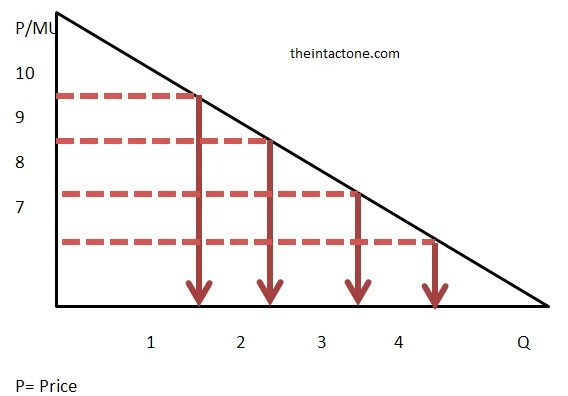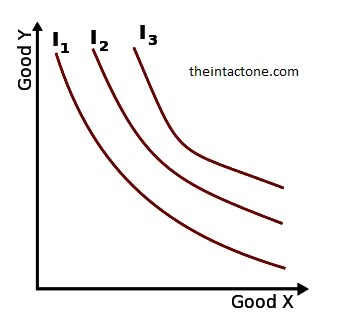# Important Differences between Cardinal Utility and Ordinal Utility

Cardinal Utility

The Cardinal Utility approach is propounded by neo-classical economists, who believe that utility is measurable, and the customer can express his satisfaction in cardinal or quantitative numbers, such as 1,2,3, and so on.

The neo-classical economist developed the theory of consumption based on the assumption that utility is measurable and can be expressed cardinally. And to do so, they have introduced a hypothetical unit called as “Utils” meaning the units of utility. Here, one Utils is equivalent to one rupee and the utility of money remains constant.

Over the passage of time, it was realized that the absolute measure of utility is not possible, i.e. it was difficult to measure the feeling of satisfaction cardinally (in numbers). Also, it was difficult to quantify the factors that cause a change in the moods of the consumer, their tastes and preferences and their likes and dislikes. Therefore, the utility is not measurable in quantitative terms. But however, it is being used as the starting point in the consumer behavior analysis.The cardinal utility approach used in analyzing the consumer behavior depends on the following assumptions:

1. Rationality

It is assumed that the consumers are rational, and they satisfy their wants in the order of their preference. This means they will purchase those commodities first which yields the highest utility and then the second highest and so on.

1. Limited Resources (Money)

The consumer has limited money to spend on the purchase of goods and services and thus this makes the consumer buy those commodities first which is a necessity.

1. Maximize Satisfaction

Every consumer aims at maximizing his/her satisfaction for the amount of money he/she spends on the goods and services.

1. Utility is cardinally Measurable

It is assumed that the utility is measurable, and the utility derived from one unit of the commodity is equal to the amount of money, which a consumer is ready to pay for it, i.e. 1 Util = 1 unit of money.

1. Diminishing Marginal Utility

This means, with the increased consumption of a commodity, the utility derived from each successive unit goes on diminishing. This law holds true for the theory of consumer behavior.

1. Marginal Utility of Money is Constant

It is assumed that the marginal utility of money remains constant irrespective of the level of a consumer’s income.

The cardinalists believe that not only the utility is measurable but also the utility derived from the consumption of different commodities are added up to realize the total utility.

Thus, the cardinal utility approach is used as a basis for explaining the consumer behavior where every individual aims at maximizing his/her utility or satisfaction for the amount of money he spends on the consumption of goods and services.

Critique of the Cardinal Approach:

There are three basic weaknesses in the cardinalist approach. The assumption of cardinal utility is extremely doubtful. The satisfaction derived from various commodities cannot be measured objectively. The attempt by Walras to use subjective units (utils) for the measurement of utility does not provide any satisfactory solution. The assump­tion of constant utility of money is also unrealistic.

As income increases the marginal utility of money changes. Thus money cannot be used as a measuring-rod since its own utility changes. Finally, the axiom of diminishing marginal utility has been ‘established’ from introspection, it is a psychological law which must be taken for granted.

Ordinal Utility

An ordinal utility function is a function representing the preferences of an agent on an ordinal scale. Ordinal utility theory claims that it is only meaningful to ask which option is better than the other, but it is meaningless to ask how much better it is or how good it is. All of the theory of consumer decision-making under conditions of certainty can be, and typically is, expressed in terms of ordinal utility.Consider a simple case where the consumer purchases only two goods, Q1 and Q2.

Ordinal utility function is:

U = f(q1, q2)

Where U is the ordinal utility number, and q1 and q2 are the quantities consumed of the two goods. Assume that U is a single-valued function of q1 and q2, and f (q1, q2) is continuous, and it has continuous first-order and second-order partial derivatives. Remember also that U has to be a regular strictly quasi-concave function of qi and q2.

Since, it shall be assumed that the consumer will desire to have more of both the goods, the partial derivatives of U (w.r.t.) q1 and q2 will be positive unless otherwise men­tioned as in some unusual cases. But remember some more points about the utility function.

First, the consumer’s utility function is not unique. Any function which is a positive monotonic transformation of his utility function may also be taken as a utility function of the consumer, for it would represent the same preference-indifference pattern.

Second, as noted, U in stands for the ordinal utility number for a particular combination of the goods. This number has no cardinal significance. It has only ordinal significance. It indicates the utility-rank of the said combination to the consumer.

Since the utility numbers have no cardinal significance, the two numbers indicating two utility-ranks of two particular combinations of goods may be, say, 2 and 3, or they may even be 2 and 300, the higher number indicating the higher utility rank of the two.

Third, the utility function is defined for a particular time period. The consumer’s optimal expenditure pattern is analysed only with respect to this period. The possibility of transferring consumption expenditure from one period to another has not been considered here.

Remember that the time period should not be so short that the desire for variety cannot be satisfied, neither should it be so long that the consumer’s tastes and the shape of his utility function might change meanwhile. Any intermediate period should be appropriate for the static theory of the consumer behaviour.

 Ordinal Utility Cardinal Utility An ordinal utility function is a function representing the preferences of an agent on an ordinal scale. Consumer derived satisfaction after consumption of products evaluated in numerical numbers Ordinal utility used qualitative approach during analysis of degree of the satisfaction. Cardinal utility used quantitative approach during analysis of degree of satisfaction. Consumer in ordinal utility rank of preference according to their derived satisfaction after consumption of products. Consumer in cardinal utility makes measurements of degree of satisfaction derived after consuming products in utils. Ordinal utility measurements promoted by modern economist Cardinal utility measurement promoted by traditional economist. Qualitative Approach Quantitative Approach Ordinal utility measurements results considered as more realistic. Cardinal utility measurement results considered not realistic in comparison with ordinal utility. Measurement  by Ranks Measurement  by Utils Indifference curve analysis used for explanation ordinal utility. Marginal utility analysis used for explanation of cardinal utility.#### intactone

View all posts by intactone →
error: Content is protected !!